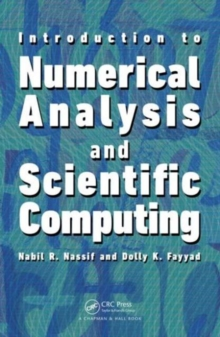Supporting your high street Find out how »
• My Account# Introduction to Numerical Analysis and Scientific Computing Hardback

#### Description

Designed for a one-semester course, Introduction to Numerical Analysis and Scientific Computing presents fundamental concepts of numerical mathematics and explains how to implement and program numerical methods.

The classroom-tested text helps students understand floating point number representations, particularly those pertaining to IEEE simple and double-precision standards as used in scientific computer environments such as MATLAB (R) version 7.

Drawing on their years of teaching students in mathematics, engineering, and the sciences, the authors discuss computer arithmetic as a source for generating round-off errors and how to avoid the use of algebraic expression that may lead to loss of significant figures.

They cover nonlinear equations, linear algebra concepts, the Lagrange interpolation theorem, numerical differentiation and integration, and ODEs.

They also focus on the implementation of the algorithms using MATLAB (R). Each chapter ends with a large number of exercises, with answers to odd-numbered exercises provided at the end of the book.

Throughout the seven chapters, several computer projects are proposed.

These test the students' understanding of both the mathematics of numerical methods and the art of computer programming.

#### Information

• Format: Hardback
• Pages: 329 pages, 115 Tables, black and white; 26 Illustrations, black and white
• Publisher: Taylor & Francis Inc
• Publication Date:
• ISBN: 9781466589483

£77.99

£68.05

on all orders

###### Pick up orders

from local bookshops